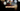# Leetcode | Solution of Length of Last Word in JavaScript

March 27th, 2020
|

In this post, we will solve the problem length of last word from leetcode and compute the time and space complexities. Let's begin.

# Problem Statement

The question can be found at leetcode length of last word problem.

The problem states that we are given a sentence of characters which include uppercase, lowercase, number, and spaces and we need to find the length of the last word in the sentence. A word is a sequence of non-space characters.

So a space i.e. " ", something like this is not a word.

Let's take an example- "I saw a cat", in this string, the last word is "cat". If we modify the string "I saw a cat ", i.e. add some extra space at the end... the last word is still "cat", spaces are not words according to the question.

# Solution

Following our discussion above, we are going to take the following steps

• First, we'll trim the sentence, it will remove extra white spaces from both ends of the string
• Next, we'll split the sentence by a space character which will give us a list of all the words
• At last, we'll check if word list has any entries, if yes, we'll return the length of the last word

We have discussed the approach, I urge you to go ahead on leetcode and give it another try.If you are here, it means something went wrong in implementation or you are just too lazy. In any case, let's see a simple implementation of the above logic.
``````var lengthOfLastWord = function (s) {
const words = s.trim().split(" ");
return words.length > 0 ? words[words.length - 1].length : 0;
};``````

Let's look at the solution.

First, we trim and split the sentence into words. Next, we check if the words length is greater than zero, we return the length of the last word, else we return zero(according to the question)

After submission, here's the result

``````Status: Accepted
Runtime: 44ms
Memory: 33MB``````

## Time and space complexity

### Time complexity

We are splitting the sentence into words which are dependent on the length of the string. So, the time complexity is O(n).

### Space complexity

We are using extra space to store words in an array, thus, space complexity would be O(n).

# Summary

So, we solved the length of last word problem and computed it's time and space complexity.

I hope you enjoyed solving this question. This is it for this one, complete source code for this post can be found on my Github Repo. Will see you in the next one.